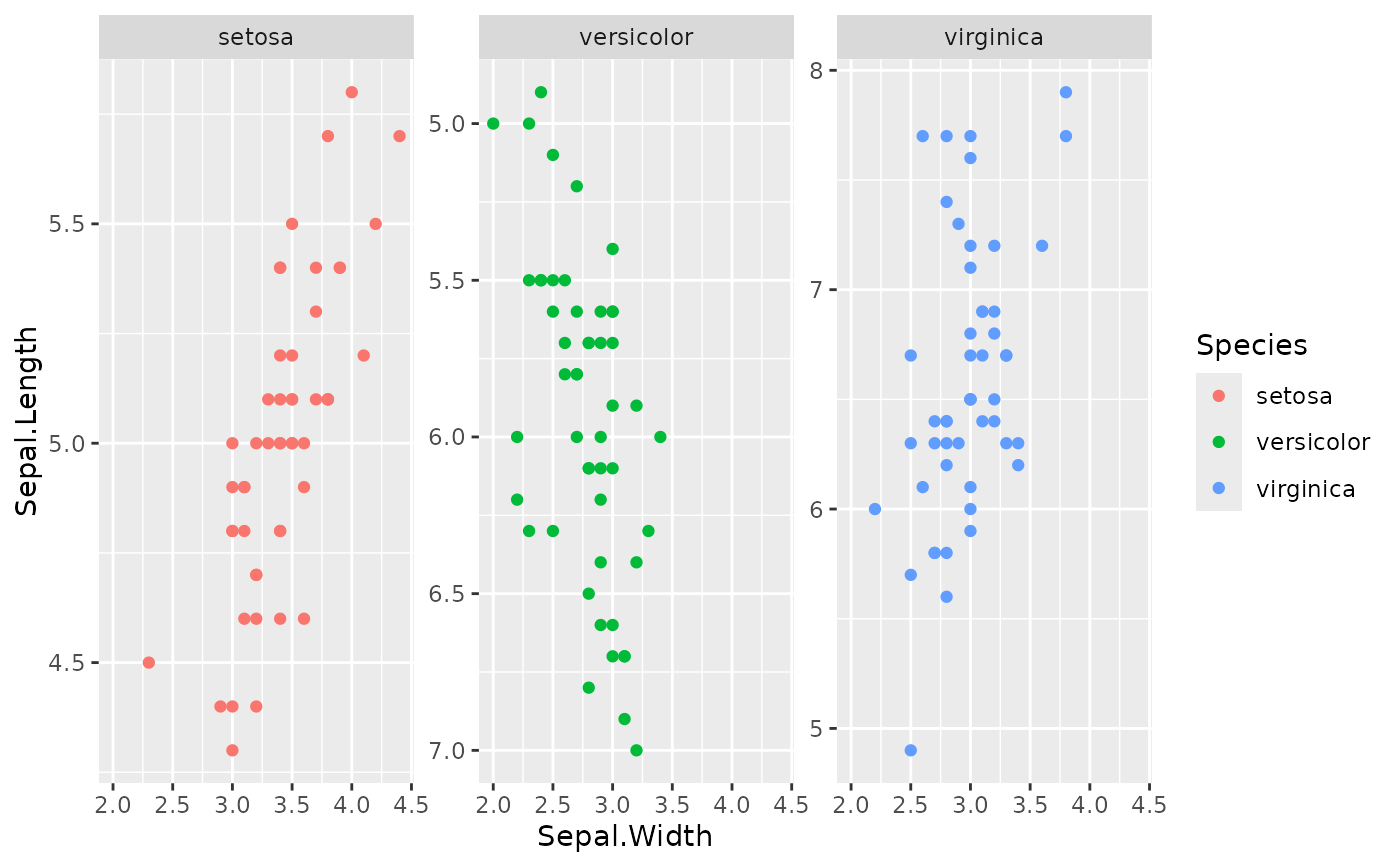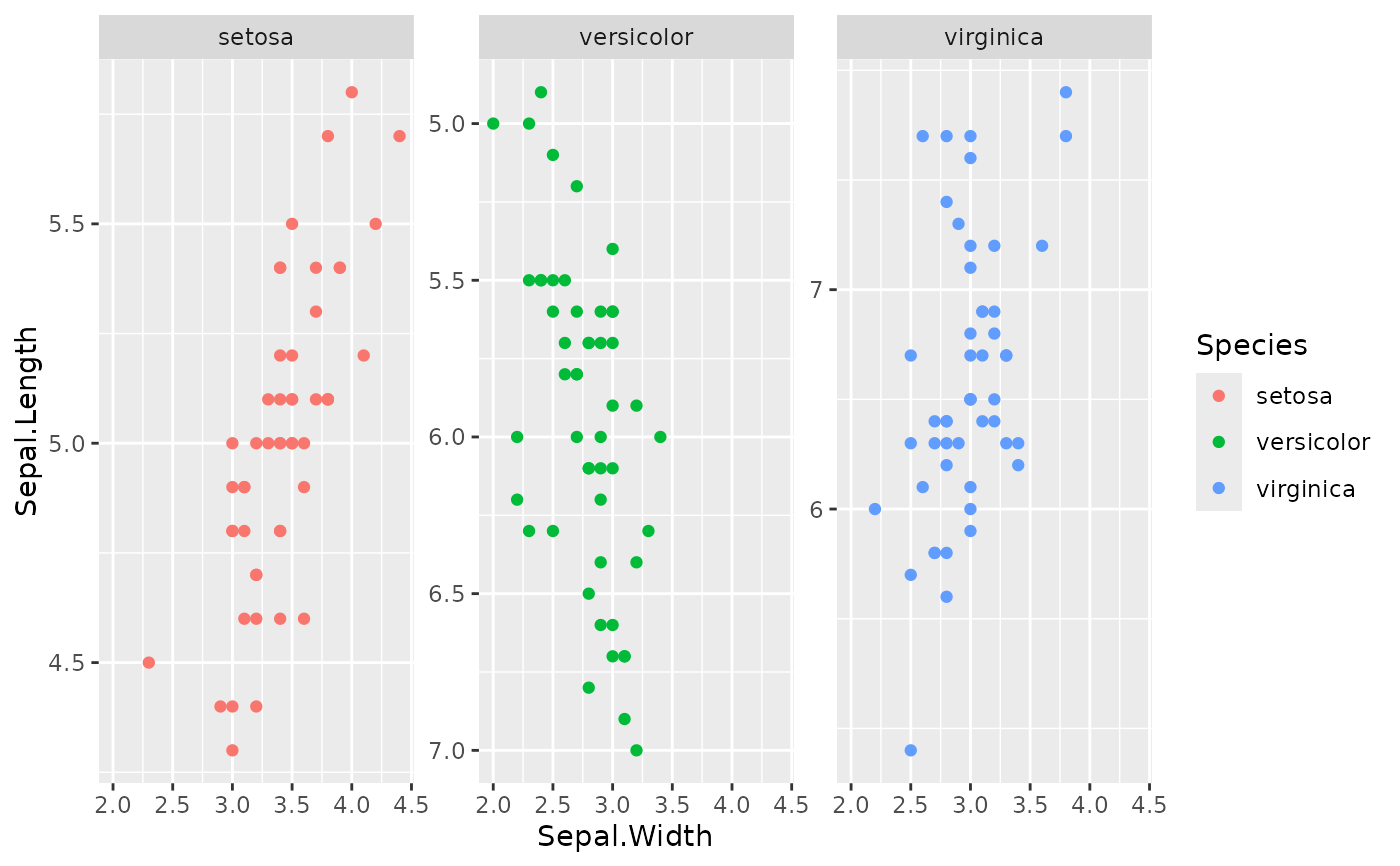This function allows the tweaking of the position scales (x and y) of individual facets. You can use it to fine-tune limits, breaks and other scale parameters for individual facets, provided the facet allows free scales.

## Usage

facetted_pos_scales(x = NULL, y = NULL)

## Arguments

x, y

A list wherein elements are either x/y position scales or NULLs. Alternatively, a list of formulae (see details).

## Value

A facetted_pos_scales object, instructing a ggplot how to adjust the scales per facet.

## Details

It is intended that this function works with both ggplot2::facet_wrap() and ggplot2::facet_grid(). For facet_wrap, the scales are used for each individual panel. For facet_grid, the scales are used for the rows and columns. Note that these facets must be used with scales = "free" or "free_x" or "free_y", depending on what scales are added.

Axis titles are derived from the first scale in the list (or the default position scale when the first list element is NULL).

### Scale transformations

It is allowed to use individual scale transformations for facets, but this functionality comes with the trade-off that the out of bounds (oob) argument for individual scales is ignored. Values that are out of bounds will be clipped. Whereas the stat part of a ggplot layer is typically calculated after scale transformations, the calculation of the stat happens before scale transformation with this function, which can lead to some awkward results. The suggested workaround is to pre-transform the data for layers with non-identity stat parts.

### Scale list input

NULLs are valid list elements and signal that the default position scale should be used at the position in the list where the NULL occurs. Since transformations are applied before facet scales are initiated, it is not recommended to use a default position (either the first in the list, or defined outside facetted_pos_scales()) scale with a transformation other than trans = "identity" (the default).

### Formula list input

The x and y arguments also accept a list of two-sided formulas. The left hand side of a formula should evaluate to a logical vector. The right hand side of the formula should evaluate to a position scale, wherein the x argument accepts x-position scales and the y argument accepts y-position scales. Notably, the left hand side of the formula is evaluated using the tidy evaluation framework, whereby the data.frame with the plot's layout is given priority over the environment in which the formula was created. As a consequence, variables (columns) that define faceting groups can be references directly.

ggplot2::scale_x_continuous() and scale_x_discrete.

## Examples

plot <- ggplot(iris, aes(Sepal.Width, Sepal.Length)) +
geom_point(aes(colour = Species)) +
facet_wrap(Species ~ ., scales = "free_y")

# Reversing the y-axis in the second panel. When providing a list of scales,
# NULL indicates to use the default, global scale
plot +
facetted_pos_scales(
y = list(NULL, scale_y_continuous(trans = "reverse"))
)# Alternative for specifying scales with formula lists. The LHS can access
# columns in the plot's layout.
plot +
facetted_pos_scales(
y = list(
Species == "virginica" ~ scale_y_continuous(breaks = c(6, 7)),
Species == "versicolor" ~ scale_y_reverse()
)
)